GATE  >  Mining Engineering - MN 2015 GATE Paper (Practice Test)

# Mining Engineering - MN 2015 GATE Paper (Practice Test) - GATE

Test Description

## 65 Questions MCQ Test GATE Past Year Papers for Practice (All Branches) - Mining Engineering - MN 2015 GATE Paper (Practice Test)

Mining Engineering - MN 2015 GATE Paper (Practice Test) for GATE 2023 is part of GATE Past Year Papers for Practice (All Branches) preparation. The Mining Engineering - MN 2015 GATE Paper (Practice Test) questions and answers have been prepared according to the GATE exam syllabus.The Mining Engineering - MN 2015 GATE Paper (Practice Test) MCQs are made for GATE 2023 Exam. Find important definitions, questions, notes, meanings, examples, exercises, MCQs and online tests for Mining Engineering - MN 2015 GATE Paper (Practice Test) below.
Solutions of Mining Engineering - MN 2015 GATE Paper (Practice Test) questions in English are available as part of our GATE Past Year Papers for Practice (All Branches) for GATE & Mining Engineering - MN 2015 GATE Paper (Practice Test) solutions in Hindi for GATE Past Year Papers for Practice (All Branches) course. Download more important topics, notes, lectures and mock test series for GATE Exam by signing up for free. Attempt Mining Engineering - MN 2015 GATE Paper (Practice Test) | 65 questions in 180 minutes | Mock test for GATE preparation | Free important questions MCQ to study GATE Past Year Papers for Practice (All Branches) for GATE Exam | Download free PDF with solutions
 1 Crore+ students have signed up on EduRev. Have you?
Mining Engineering - MN 2015 GATE Paper (Practice Test) - Question 1

### Q. 1 – Q. 5 carry one mark each. Q. Choose the appropriate word/pharase, out of the four options given below, to complete the following sentence: Apparent lifelessness _________________ dormant life.

Mining Engineering - MN 2015 GATE Paper (Practice Test) - Question 2

### fill in the blank with the correct idiom/ pharase That boy from the town was a _________________ in the sleepy village.

Mining Engineering - MN 2015 GATE Paper (Practice Test) - Question 3

### Choose the statement where underlined word is used correctly

Mining Engineering - MN 2015 GATE Paper (Practice Test) - Question 4

Tanya is older than Eric

Cliff is older than Tanya

Eric is older that cliff

if the first two statements are true, the the third statement is :

Mining Engineering - MN 2015 GATE Paper (Practice Test) - Question 5

Five teams have to compete in a league, with every team playing every other team exactly once, before going to the next round. ow many matches will have to be held to complete the league round of matches?

Mining Engineering - MN 2015 GATE Paper (Practice Test) - Question 6

Q. 6 – Q. 10 carry two mark each.

Q.

Select the appropriate option in place of underlines part of the sentence

Increased productivity necessary reflects greater efforts made by the employees.

Mining Engineering - MN 2015 GATE Paper (Practice Test) - Question 7

Given below are two statements followed by two conclusion. Assuming these statements to be true, decide which one logically follows.

Statement:

1  No manager is a leader

Conclusions:

1.  No manager is an executive

2.  No executive is a manager.

*Answer can only contain numeric values
Mining Engineering - MN 2015 GATE Paper (Practice Test) - Question 8

In the fiven figure angle Q is a right angle, PS:QS=3:1, RT: QT =5:2 and PU: UR = 1:1 if area of triangle QTS is 20 cm2, then the area of triangle PQR in CMis __________.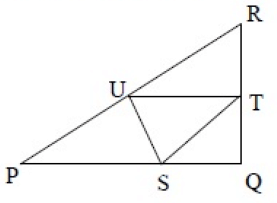(Important : you should answer only the numeric value)

Mining Engineering - MN 2015 GATE Paper (Practice Test) - Question 9

Right triangle PQR is to be constructed in the xy - plane so that the right angle is at P and line PR is parallel to the x-axis. Te x and y coordinates of P, Q and R are to be integers that satisfy the inequalities: -4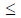x5 and  616. How many diffrent traingle xould be constructed with these properties ?

Mining Engineering - MN 2015 GATE Paper (Practice Test) - Question 10

A coin is tosssed thrice. Let X be the event that head occurs in each of the first two tosses. Let Y be the event that a tail occurs on the third toss. Let Z be the event that two tails occur in three tosses. Based on the above information, Which one of the following statements is TRUE?

Mining Engineering - MN 2015 GATE Paper (Practice Test) - Question 11

Q. 1 – Q. 25 carry one mark each.

Q.

Out of the support categories given for an underground coal mine, identify the ‘active support’.

Mining Engineering - MN 2015 GATE Paper (Practice Test) - Question 12

Massive sandstone in immediate roof delays the local fall in goaf of a coal mine. Under this
condition, crushing of the pillars at outbye side is called

*Answer can only contain numeric values
Mining Engineering - MN 2015 GATE Paper (Practice Test) - Question 13

A back sight on a bench mark of RL 100.00 m on the floor of a tunnel is 3.25 m. The inverse staff
reading on a roof station of the tunnel is 1.25 m. The RL of the roof station in m is ________

Mining Engineering - MN 2015 GATE Paper (Practice Test) - Question 14

The angle in degrees at which a ridge line intersects contours is

Mining Engineering - MN 2015 GATE Paper (Practice Test) - Question 15

In a drum hoisting system through a vertical shaft, overwinding is prevented by

Mining Engineering - MN 2015 GATE Paper (Practice Test) - Question 16

The temperature of a parcel of air decreases from 30.2o C to 28.9o C as it rises from an altitude of
20 m to 120 m. The lapse rate for the atmosphere is

Mining Engineering - MN 2015 GATE Paper (Practice Test) - Question 17

The excess pore pressure in backfill material in a cut-and-fill stope leads to

Mining Engineering - MN 2015 GATE Paper (Practice Test) - Question 18

The primary purpose of cut holes for blasting in an underground drivage is to

Mining Engineering - MN 2015 GATE Paper (Practice Test) - Question 19

In a triangle ABC, the bearings of the sides AB, BC, and CA are 600 , 1300, and 2700 respectively.
The interior angles A, B, and C in degrees respectively are

Mining Engineering - MN 2015 GATE Paper (Practice Test) - Question 20

In a binomial distribution, the probability of success p →0 and number of trials n → ∞ such that
λ = np approaches to a finite value. The variance of the distribution is

*Answer can only contain numeric values
Mining Engineering - MN 2015 GATE Paper (Practice Test) - Question 21

For a function f (x) , it is given that f (0) = 2 and f' (0) = 4 . Ignoring all other higher order
derivative terms, the value of f (0.5) is ________

(Important : you should answer only the numeric value)

Mining Engineering - MN 2015 GATE Paper (Practice Test) - Question 22

The two sides of a parallelogram are given by the vectors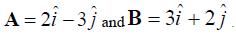The
area of the parallelogram is

Mining Engineering - MN 2015 GATE Paper (Practice Test) - Question 23

In a BOD test, 5 ml of wastewater is diluted with pure water to fill a 300 ml BOD bottle. The
initial and final dissolved oxygen contents of the mix are 9.0 mg/l and 7.0 mg/l respectively. The
BOD of the wastewater, in mg/l, is

*Answer can only contain numeric values
Mining Engineering - MN 2015 GATE Paper (Practice Test) - Question 24

A force of 50 N is applied to a wrench as shown in the figure. The magnitude of the moment in
N-mm of this force about the point P is ________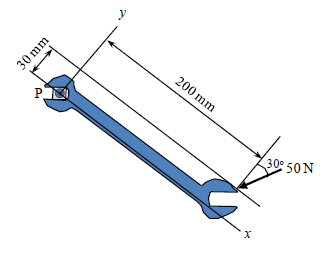Mining Engineering - MN 2015 GATE Paper (Practice Test) - Question 25

Dilatancy of rock is associated with

*Answer can only contain numeric values
Mining Engineering - MN 2015 GATE Paper (Practice Test) - Question 26

A bord and pillar panel having square pillars is designed for 30% extraction during development. If
the gallery width is 5 m, the side of the pillar in m is ________

Mining Engineering - MN 2015 GATE Paper (Practice Test) - Question 27

Low shock and high gas pressure explosive is generally used for blasting of

Mining Engineering - MN 2015 GATE Paper (Practice Test) - Question 28

The covariance of copper grade for a certain lag distance in an ore body is 6.0 (%)2. If the sill is
10 (%)2, the semivariogram for the same lag distance in (%)2 is

Mining Engineering - MN 2015 GATE Paper (Practice Test) - Question 29

The matrix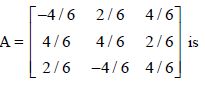*Answer can only contain numeric values
Mining Engineering - MN 2015 GATE Paper (Practice Test) - Question 30

A gas mixture contains CH4, C2H6 and H2 with respective concentrations of 75%, 15% and 10% by
volume. The lower explosibility limit of CH4, C2H6 and H2 are 5.0%, 3.3% and 4.2% respectively.
The lower explosibility limit of the gas mixture, in percentage, is ________

*Answer can only contain numeric values
Mining Engineering - MN 2015 GATE Paper (Practice Test) - Question 31

Intake air containing 0.2% methane enters a section of an underground mine where emission rate of
methane is 0.05 m3/s. Assuming that the threshold limit value of methane is 1.25%, the minimum
quantity of fresh air required in m3/s is ________

Mining Engineering - MN 2015 GATE Paper (Practice Test) - Question 32

In a fully mechanised bord and pillar mining system, winning of coal and its transportation from the
face is commonly carried out with the combination of

*Answer can only contain numeric values
Mining Engineering - MN 2015 GATE Paper (Practice Test) - Question 33

An underground coal mine employing 1200 persons experiences 2 fatal injuries, 6 serious injuries
and 8 reportable injuries during the year 2013. The total injury rate per 1000 persons employed for
the year is ________

Mining Engineering - MN 2015 GATE Paper (Practice Test) - Question 34

In self-contained chemical-oxygen self-rescuer, oxygen is produced by

*Answer can only contain numeric values
Mining Engineering - MN 2015 GATE Paper (Practice Test) - Question 35

The failure data of an equipment follows an exponential distribution. If the mean time between
failures is 3000 hours, the reliability of the equipment for 750 hours is ________

Mining Engineering - MN 2015 GATE Paper (Practice Test) - Question 36

Q. 36 – Q. 65 carry two marks each.

Q.

In a 4.2 m wide and 3.0 m high gallery in a coal seam, twelve shot holes are blasted per round. The
holes are charged with 2 explosive cartridges of 435 g each. If the powder factor of the blast is 2.2
tonne/kg and specific gravity of coal is 1.4, the pull per round of blast in m is

*Answer can only contain numeric values
Mining Engineering - MN 2015 GATE Paper (Practice Test) - Question 37

The stadia readings with horizontal sight on a vertical staff held at 50 m from a tacheometer are
1.285 m and 1.780 m. The focal length of the object glass is 25 cm, and the distance between the
object glass and the vertical axis of the tacheometer is 15 cm. The stadia interval in mm is _______

Mining Engineering - MN 2015 GATE Paper (Practice Test) - Question 38

In a shortwall panel, coal is extracted from the face by a continuous miner having rate of production
30 tonne/h. Coal having specific gravity of 1.4 is transported by shuttle cars of capacity 0.9 m3
each to a feeder breaker located at 60 m from the face. If the average speed of the LHD is 0.5 m/s,
production of the continuous miner is

*Answer can only contain numeric values
Mining Engineering - MN 2015 GATE Paper (Practice Test) - Question 39

Vertical photographs of an area lying 500 m above the mean sea level are to be taken at a scale of
1:20000 from an aircraft. If the camera has a focal length of 210 mm, the flying height of the
aircraft above the mean sea level in m is ________

(Important : you should answer only the numeric value)

Mining Engineering - MN 2015 GATE Paper (Practice Test) - Question 40

Match the following locations with support types in coal mines.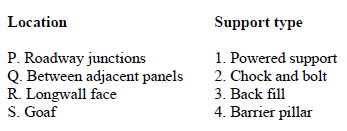Mining Engineering - MN 2015 GATE Paper (Practice Test) - Question 41

The value of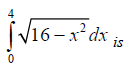*Answer can only contain numeric values
Mining Engineering - MN 2015 GATE Paper (Practice Test) - Question 42

A rectangular field of area 20000 m2 is to be divided into 6 different plots by fencing as shown in
the figure. The value of L in m for which the total length of fencing becomes minimum is _______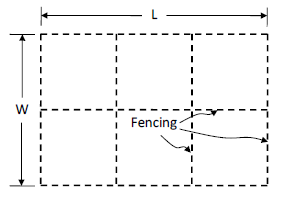Mining Engineering - MN 2015 GATE Paper (Practice Test) - Question 43

Match the following for a drilling system.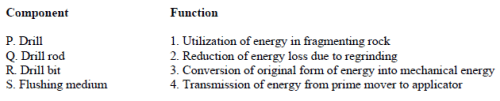*Answer can only contain numeric values
Mining Engineering - MN 2015 GATE Paper (Practice Test) - Question 44

For the ventilation system shown, the combined resistance of the trunk airways and the shafts is
2.2 Ns2m-8. The resistances of splits A and B are 0.5 Ns2m-8 and 0.8 Ns2m-8 respectively. A
regulator of size 2.0 m2 is placed in split A. Considering the fan generates a pressure of 1000 Pa,
the air flow in m3/s in split B is ________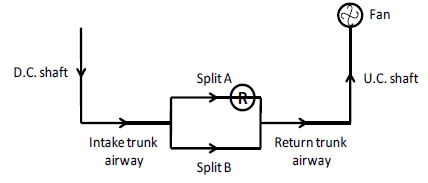*Answer can only contain numeric values
Mining Engineering - MN 2015 GATE Paper (Practice Test) - Question 45

A mine fan running at 300 rpm delivers 150 m3/s of air at a pressure of 900 Pa. Fan and motor
efficiencies are 75% and 90% respectively. If the fan speed is reduced to 250 rpm, the saving in
electric power input to the motor in kW is ________

*Answer can only contain numeric values
Mining Engineering - MN 2015 GATE Paper (Practice Test) - Question 46

Subsidence profile function, s(x), along the lateral cross-section over a flat longwall panel is given
as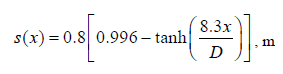where x = distance (m) from the inflection point and D = depth (m) of the seam. Considering that
the inflection point lies vertically above the edge of the panel, the angle of draw in degrees for a
depth of 250 m is ________

*Answer can only contain numeric values
Mining Engineering - MN 2015 GATE Paper (Practice Test) - Question 47

A goaf void of 250 m3 is filled in 3 hours by hydraulic sand stowing method. Density of the sand is
2.6 tonne/m3. If the filling factor of goaf void is 0.9 and sand to water ratio in the stowing mixture
is 1.0 tonne to 1.1 m3 , the stowing rate in m3/h is ________

*Answer can only contain numeric values
Mining Engineering - MN 2015 GATE Paper (Practice Test) - Question 48

A single-acting reciprocating pump delivers 0.018 m3/s of water when running at 45 cycles per
minute. The piston diameter is 300 mm and stroke length is 400 mm. The volumetric efficiency of
the pump in % is ________

Mining Engineering - MN 2015 GATE Paper (Practice Test) - Question 49

Match the method of mining with strength of orebody, type of support and orebody geometry.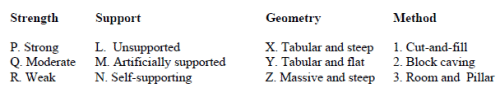*Answer can only contain numeric values
Mining Engineering - MN 2015 GATE Paper (Practice Test) - Question 50

A mine air sample contains CH4, CO, H2, N2 and O2. The mine air analysis using Haldane apparatus
gives the following results expressed in percentage of total sample volume.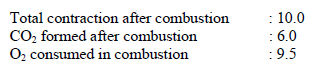The percentage of CH4 in the sample analysed is ________

*Answer can only contain numeric values
Mining Engineering - MN 2015 GATE Paper (Practice Test) - Question 51

The initial investment for a small scale mining project is Rs. 5.0 crore. Annual cash inflow for a life
period of 4 years is given below.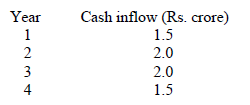The net present value of the project at an annual discount rate of 10% in Rs. crore is ________

Mining Engineering - MN 2015 GATE Paper (Practice Test) - Question 52

Given the following linear programming problem,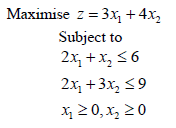the corner point feasible solution in terms of (x1,x2) is

*Answer can only contain numeric values
Mining Engineering - MN 2015 GATE Paper (Practice Test) - Question 53

The 3-period torque-time diagram of a statically balanced hoist is shown in the figure.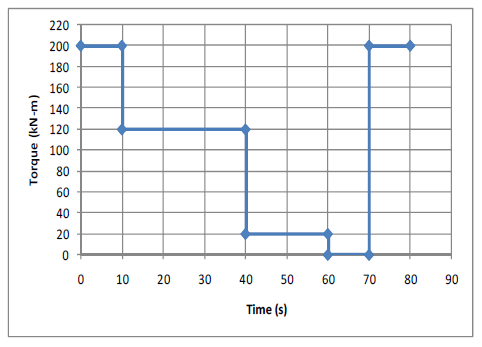The rms torque for the motor in kN-m is ________

*Answer can only contain numeric values
Mining Engineering - MN 2015 GATE Paper (Practice Test) - Question 54

Airborne PM10 concentration in a residential area is monitored for 24 hours by a respirable dust
sampler. Initial and final weights of the filter paper are 2.3125 g and 2.6996 g respectively. The
average airflow rate during sampling is 1.2 m3/min. The PM10 concentration of the area in
μg m-3 is ______

*Answer can only contain numeric values
Mining Engineering - MN 2015 GATE Paper (Practice Test) - Question 55

The assignment problem given requires four different jobs to be done on four different machines.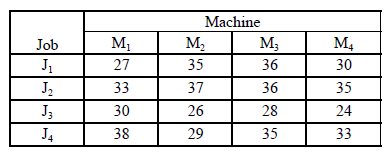The minimum cost of assignment is ________

Mining Engineering - MN 2015 GATE Paper (Practice Test) - Question 56

Acceleration of a particle moving in a straight line is expressed by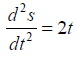where, s denotes distance (m) and t, time (s). At time t = 0, the distance and velocity of the particle
are 0 m and 3 m/s respectively. The distance travelled by the particle in m after 3 s is

*Answer can only contain numeric values
Mining Engineering - MN 2015 GATE Paper (Practice Test) - Question 57

Rock bolts have length L = (150 + X) cm, where X is a random variable with probability density
function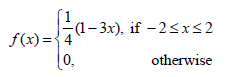If 95% of the bolt lengths (L) lie in the interval 150 - c cm to 150 + c cm, the value of c is _______

*Answer can only contain numeric values
Mining Engineering - MN 2015 GATE Paper (Practice Test) - Question 58

The properties for a bivariate distribution of two random variables X and Y are given below.

E(X) = 24, E(Y ) = 36, E(X2 ) = 702, E(Y2 ) =1524, E(XY) =1004

The correlation coefficient between X and Y is ________

Mining Engineering - MN 2015 GATE Paper (Practice Test) - Question 59

Biaxial stresses at a point inside a pillar are shown in the figure.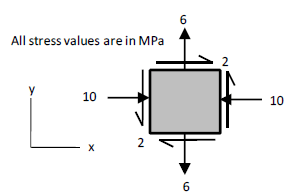The magnitude of the maximum shear stress in MPa and its direction with the x-axis in degrees at
the same point respectively are

*Answer can only contain numeric values
Mining Engineering - MN 2015 GATE Paper (Practice Test) - Question 60

A circular tunnel is constructed in a biaxial far field stress (vertical stress p0 and horizontal stress
Kp0 ) as shown in the figure.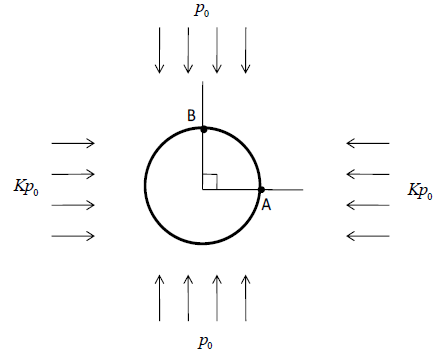If the ratio of the tangential stress measured at the boundary points A and B is 3:1, the value of K is
_______

(Important : you should answer only the numeric value)

Mining Engineering - MN 2015 GATE Paper (Practice Test) - Question 61

Peak particle velocity (PPV) at points A and B are measured for a blast pattern as shown in the
figure.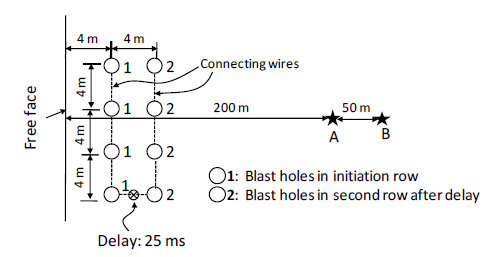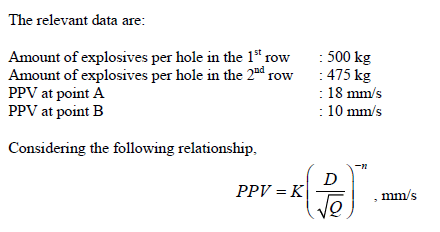where D (in m) denotes the distance from the blast row to the measuring point and Q (in kg),
maximum charge per delay. The site constants K and n respectively are

Mining Engineering - MN 2015 GATE Paper (Practice Test) - Question 62

Copper ore of average grade 0.65% is mined, milled, smelted and then refined. The following
information is available: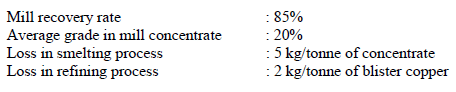The amount of refined copper obtained per tonne of ore in kg is

*Answer can only contain numeric values
Mining Engineering - MN 2015 GATE Paper (Practice Test) - Question 63

The ratio of horizontal to vertical in-situ stresses, K, at a mine field varies with depth, D (in m) as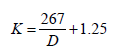If the unit weight of overburden rock is 25 kN/m3, the horizontal stress in MPa at a depth of 400 m
is ________

*Answer can only contain numeric values
Mining Engineering - MN 2015 GATE Paper (Practice Test) - Question 64

A coal seam of 2 m thickness is extracted by a longwall retreating panel with face length of 120 m.
Web depth of the shearer is 0.6 m. Average manpower in the longwall face in a shift is 20. The
specific gravity of in-situ coal is 1.4. If the shearer makes 4 full-face cuts in 3 shifts, the face OMS
in tonne is ________

*Answer can only contain numeric values
Mining Engineering - MN 2015 GATE Paper (Practice Test) - Question 65

A loaded dumper of total mass 75 tonne, having wheel diameter 1250 mm, runs on a haul road
which offers an average specific rolling resistance of 260 N/tonne. The engine develops an axle
torque of 15 kN-m. The starting acceleration of the dumper in m/s2 is ________

## GATE Past Year Papers for Practice (All Branches)

407 docs|127 tests
Information about Mining Engineering - MN 2015 GATE Paper (Practice Test) Page
In this test you can find the Exam questions for Mining Engineering - MN 2015 GATE Paper (Practice Test) solved & explained in the simplest way possible. Besides giving Questions and answers for Mining Engineering - MN 2015 GATE Paper (Practice Test), EduRev gives you an ample number of Online tests for practice

## GATE Past Year Papers for Practice (All Branches)

407 docs|127 tests(Scan QR code)